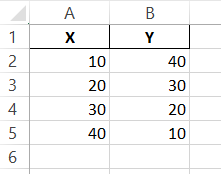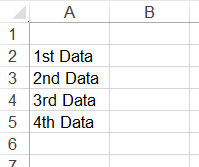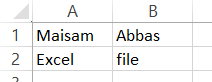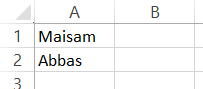# Python Export to Excel

Muhammad Maisam Abbas Jan 30, 2023 Jan 15, 2022

This tutorial will demonstrate different methods to write tabular data to an excel file in Python.

## Export Data to Excel With the `DataFrame.to_excel()` Function in Python

If we want to write tabular data to an Excel sheet in Python, we can use the `to_excel()` function in Pandas `DataFrame`.

A pandas `DataFrame` is a data structure that stores tabular data. The `to_excel()` function takes two input parameters: the file’s name and the sheet’s name. We must store our data inside a pandas `DataFrame` and then call the `to_excel()` function to export that data into an Excel file.

We need to have the pandas library already installed on our system for this method to work. The command to install the `pandas` library is given below.

``````pip install pandas
``````

A working demonstration of this approach is given below.

``````import pandas as pd
list1 = [10,20,30,40]
list2 = [40,30,20,10]
col1 = "X"
col2 = "Y"
data = pd.DataFrame({col1:list1,col2:list2})
data.to_excel('sample_data.xlsx', sheet_name='sheet1', index=False)
``````

`sample_data.xlsx` file:In the above code, we exported the data inside `list1` and `list2` as columns into the `sample_data.xlsx` Excel file with Python’s `to_excel()` function.

We first stored the data inside both lists into a pandas `DataFrame`. After that, we called the `to_excel()` function and passed the names of our output file and the sheet.

Keep in mind that this method will only work as long as the length of both lists is equal. If the lengths aren’t equal, we can compensate for the missing values by filling the shorter list with the `None` value.

This is the easiest method to write data to an Excel-compatible file in Python.

## Export Data to Excel With the `xlwt` Library in Python

The `xlwt` library is used to write data into old spreadsheets compatible with Excel versions from 95 to 2003 in Python. It is the standard way for writing data to Excel files in Python.

It is also fairly simple and gives us more control over the Excel file than the previous method. We can create an object of the `xlwt.Workbook` class and call the `.add_sheet()` function to create a new sheet in our workbook.

We can then use the `write()` method to write our data. This `write()` function takes the row index (starting from 0), the column index (also starting from 0), and the data to be written as input parameters.

We need to install the `xlwt` library on our machine for this method to work. The command to install the library is given below.

``````pip install xlwt
``````

A brief working example of this method is given below.

``````import xlwt
from xlwt import Workbook

wb = Workbook()

#sheet1.write(row,col, data, style)
sheet1.write(1, 0, '1st Data')
sheet1.write(2, 0, '2nd Data')
sheet1.write(3, 0, '3rd Data')
sheet1.write(4, 0, '4th Data')

wb.save('sample_data2.xls')
``````

`sample_data2.xls` file:In Python, we wrote data to the `sample_data2.xls` file with the `xlwt` library.

We first created an object of the `Workbook` class. Using this object, we created a sheet with the `add_sheet()` method of the `Workbook` class.

We then wrote our data into the newly created sheet with the `write()` function. Lastly, when all the data has been properly written to its specified index, we saved the workbook into an Excel file with the `save()` function of the `Workbook` class.

This is a pretty straightforward approach, but the only drawback is that we have to remember the row and column index for each cell in our file. We can’t just use `A1` and `A2` indices. Another disadvantage of this approach is that we can only write files with the `.xls` extension.

## Export Data to Excel With the `openpyxl` Library in Python

Another method that can be used to write data to an Excel-compatible file is the `openpyxl` library in Python.

This approach addresses all the drawbacks of the previous methods. We don’t need to remember the exact row and column indices for each data point. Simply specify our cells like `A1` or `A2` in the `write()` function.

Another cool advantage of this approach is that it can be used to write files with the new `.xlsx` file extensions, which wasn’t the case in the previous approach. This method works just like the previous one.

The only difference here is that we have to initialize each cell in addition to a sheet with the `cell(row,col)` method in the `openpyxl` library.

The `openpyxl` is also an external library. We need to install this library for this method to work properly. The command to install the `openpyxl` library on our machine is below.

``````pip install openpyxl
``````

A simple working demonstration of this approach is given below.

``````import openpyxl
my_wb = openpyxl.Workbook()
my_sheet = my_wb.active
c1 = my_sheet.cell(row = 1, column = 1)
c1.value = "Maisam"
c2 = my_sheet.cell(row= 1 , column = 2)
c2.value = "Abbas"
c3 = my_sheet['A2']
c3.value = "Excel"
# for B2: column = 2 & row = 2.
c4 = my_sheet['B2']
c4.value = "file"
my_wb.save("sample_data3.xlsx")
``````

`sample_data3.xlsx` file:In the above code, we wrote data to the `sample_data3.xlsx` Excel file with the `openpyxl` library in Python.

We first created an object of the `Workbook` class. We created a sheet with the `Workbook.active` using this object. We also created a cell object with `my_sheet.cell(row = 1, column = 1)`.

Instead of writing the exact row and column number, we can also specify the cell name like `A1`. We can then assign our newly created cell value with `c1.value = "Maisam"`.

Lastly, when all the data has been properly written to its specified index, we saved the workbook into an Excel file with the `save()` function of the `Workbook` class.

## Export Data to Excel With the `XlsWriter` Library in Python

Another great and simple way to write data to an Excel-compatible file is the `XlsWriter` library in Python.

This library gives us much more control over our output file than any previous methods mentioned above. This library also supports the latest Excel compatible file extensions like `xlsx`.

To write data to an Excel file, we first have to create an object of the `Workbook` class by providing the constructor’s file name as an input parameter. We then have to create a sheet with the `add_worksheet()` function in the `Workbook` class.

After adding a sheet, we can write data with the `sheet.write(cell, data)` function. This `sheet.write()` function takes two parameters: the cell’s name and the data to be written.

After writing all the data to the sheet, we need to close our workbook with the `close()` method inside the `Workbook` class.

The `XlsWriter` is an external library and does not come pre-installed with Python. We first have to install the `XlsWriter` library on our machine for this method to work. The command to install the `XlsWriter` library is given below.

``````pip install XlsxWriter
``````

A working demonstration of this approach is shown below.

``````import xlsxwriter

workbook = xlsxwriter.Workbook('sample_data4.xlsx')

sheet.write('A1', 'Maisam')
sheet.write('A2', 'Abbas')

workbook.close()
``````

`sample_data4.xlsx` file:We wrote data to the `sample_data4.xlsx` Excel file with Python’s `xlswriter` library in the above code.

Maisam is a highly skilled and motivated Data Scientist. He has over 4 years of experience with Python programming language. He loves solving complex problems and sharing his results on the internet.